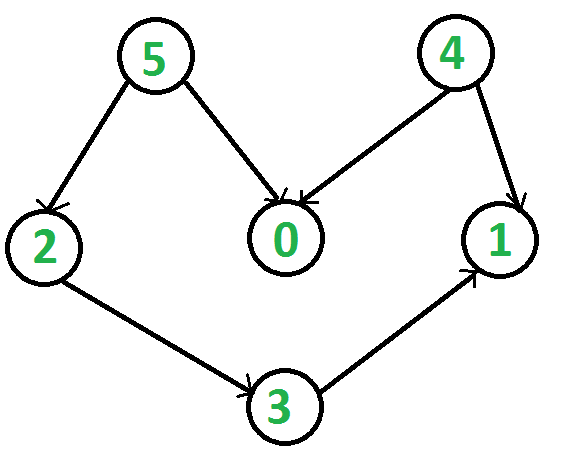Related Articles
Print all possible paths in a DAG from vertex whose indegree is 0
• Last Updated : 07 Jul, 2020

Given a Directed Acyclic Graph (DAG), having N vertices and M edges, The task is to print all path starting from vertex whose in-degree is zero.

Indegree of a vertex is the total number of incoming edges to a vertex.

Example:

Input: N = 6, edges[] = {{5, 0}, {5, 2}, {4, 0}, {4, 1}, {2, 3}, {3, 1}}
Output: All possible paths:
4 0
4 1
5 0
5 2 3 1
Explaination:
The given graph can be represented as:There are two vertices whose indegree are zero i.e vertex 5 and 4, after exploring these vertices we got the fallowing path:
4 -> 0
4 -> 1
5 -> 0
5 -> 2 -> 3 -> 1
Input: N = 6, edges[] = {{0, 5}}
Output: All possible paths:
0 5
Explaination:
There will be only one possible path in the graph.

## Recommended: Please try your approach on {IDE} first, before moving on to the solution.

Approach:

The above problem can be solve by exploring all indegree 0 vertices and printing all vertices in path until a vertex having outdegree 0 is encountered. Follow these steps to print all possible paths:

1. Create a boolean array indeg0 which store a true value for all those vertex whose indegree is zero.
2. Apply DFS on all those vertex whose indegree is 0.
3. Print all path starting from a vertex whose indegree is 0 to a vertex whose outdegrees are zero.

Illustration:
For the above graph:
indeg0[] = {False, False, False, False, True, True}
Since indeg = True, so appling DFS on vertex 4 and printing all path terminating to 0 outdegree vertex are as follow:
4 -> 0
4 -> 1

Also indeg = True, so appling DFS on vertex 5 and printing all path terminating to 0 outdegree vertex are as follow:
5 -> 0
5 -> 2 -> 3 -> 1

Here is the implementation of the above approach:

## Python3

 `# Python program to print all``# possible paths in a DAG`` ` `# Recursive function to print all paths``def` `dfs(s):``    ``# Append the node in path``    ``# and set visited``    ``path.append(s)``    ``visited[s] ``=` `True`` ` `    ``# Path started with a node``    ``# having in-degree 0 and``    ``# current node has out-degree 0,``    ``# print current path``    ``if` `outdeg0[s] ``and` `indeg0[path[``0``]]:``        ``print``(``*``path)`` ` `    ``# Recursive call to print all paths``    ``for` `node ``in` `adj[s]:``        ``if` `not` `visited[node]:``            ``dfs(node)`` ` `    ``# Remove node from path``    ``# and set unvisited``    ``path.pop()``    ``visited[s] ``=` `False`` ` ` ` `def` `print_all_paths(n):``    ``for` `i ``in` `range``(n):`` ` `        ``# for each node with in-degree 0``        ``# print all possible paths``        ``if` `indeg0[i] ``and` `adj[i]:``            ``path ``=` `[]``            ``visited ``=` `[``False``] ``*` `(n ``+` `1``)``            ``dfs(i)`` ` ` ` `# Driver code``from` `collections ``import` `defaultdict`` ` `n ``=` `6``# set all nodes unvisited``visited ``=` `[``False``] ``*` `(n ``+` `1``)``path ``=` `[]`` ` `# edges = (a, b): a -> b``edges ``=` `[(``5``, ``0``), (``5``, ``2``), (``2``, ``3``),``         ``(``4``, ``0``), (``4``, ``1``), (``3``, ``1``)]`` ` `# adjacency list for nodes``adj ``=` `defaultdict(``list``)`` ` `# indeg0 and outdeg0 arrays``indeg0 ``=` `[``True``]``*``n``outdeg0 ``=` `[``True``]``*``n`` ` `for` `edge ``in` `edges:``    ``u, v ``=` `edge[``0``], edge[``1``]``    ``# u -> v``    ``adj[u].append(v)`` ` `    ``# set indeg0[v] <- false``    ``indeg0[v] ``=` `False`` ` `    ``# set outdeg0[u] <- false``    ``outdeg0[u] ``=` `False`` ` `print``(``'All possible paths:'``)``print_all_paths(n)`
Output:
```All possible paths:
4 0
4 1
5 0
5 2 3 1
```

Time Complexity: O (N + E)2
Auxiliary Space: O (N)

Attention reader! Don’t stop learning now. Get hold of all the important DSA concepts with the DSA Self Paced Course at a student-friendly price and become industry ready.  To complete your preparation from learning a language to DS Algo and many more,  please refer Complete Interview Preparation Course.

My Personal Notes arrow_drop_up